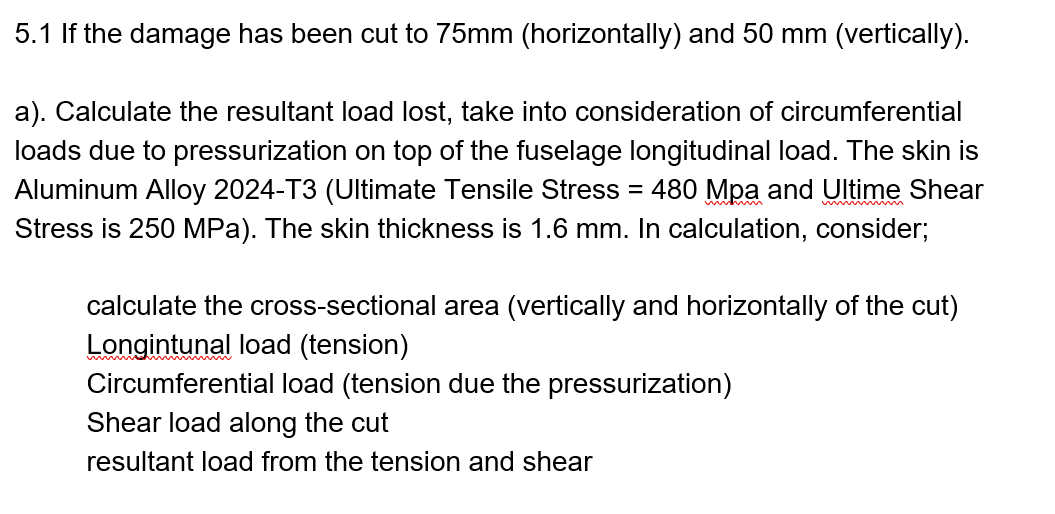Home / Expert Answers / Civil Engineering / 5-1-if-the-damage-has-been-cut-to-75-mathrm-mm-horizontally-and-50-mathrm-mm-pa858

# (Solved): $$5.1$$ If the damage has been cut to $$75 \mathrm{~mm}$$ (horizontally) and $$50 \mathrm{~mm ...\( 5.1$$ If the damage has been cut to $$75 \mathrm{~mm}$$ (horizontally) and $$50 \mathrm{~mm}$$ (vertically). a). Calculate the resultant load lost, take into consideration of circumferential loads due to pressurization on top of the fuselage longitudinal load. The skin is Aluminum Alloy 2024-T3 (Ultimate Tensile Stress = $$480 \mathrm{Mpa}$$ and Ultime Shear Stress is $$250 \mathrm{MPa})$$. The skin thickness is $$1.6 \mathrm{~mm}$$. In calculation, consider; calculate the cross-sectional area (vertically and horizontally of the cut) Longintunal load (tension) Circumferential load (tension due the pressurization) Shear load along the cut resultant load from the tension and shear

We have an Answer from Expert